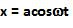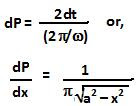## Sunday, June 6, 2010

### Irodov Problem 4.9The figure depicts the graph of position x versus time t for a oscillating particle.
Suppose that the particle is located at a position x at a certain time t. We know that the position of the particle is given by,hence, the speed (not velocity) of the particle is given by,The time dt taken by the particle to move a distance dx starting from x is then given by,As seen in the figure, the particle crosses the interval x to x+dx, twice. Once on the onward journey towards the extreme end and once on the return journey. It this spends 2dt time within the interval dx. The probability dP of finding the particle within the interval x to x+dx is the time 2dt divided by the total time period of the oscillation and is given by,1.2.3.